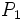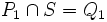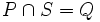# Going-down subring

Jump to: navigation, search
This article defines a property that can be evaluated for a unital subring in a commutative unital ring: given any commutative unital ring and a subring thereof, the property is either true or false for the pair
View a complete list of such properties

## Definition

### Definition with symbols

Suppose$S$ is a unital subring of a commutative unital ring$R$. We say that$S$ is a going-down subring if given prime idaels$Q \subseteq Q_1$ of$S$ and a prime ideal$P_1$ of$R$ lying over$Q_1$ (viz$P_1 \cap S = Q_1$, there exists a prime$P$ lying over$Q$ (viz$P \cap S = Q$ and contained in$P_1$.

## Facts

If$S$ is a normal domain and$R$ is an integral extension of$S$, then$R$ is a going-down subring.